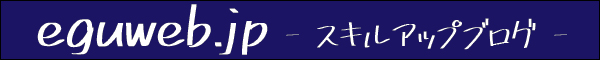## List of APIs that can be used with the QUERY function

So far, we have practiced how to use and apply the QUERY function.

``QUERY(データ, クエリ, [見出し])``

The QUERY function uses the query language of the Google Visualization API to query the entire data.

This time, I will summarize the “Query language of the Google Visualization API” as a summary so far.

Here is the official document ▼

Query Language Reference (Version 0.7) | Charts | Google Developers

## Language Clauses

Query functions can use the following query language.

You can do so many things!

## SELECT * (column selection)

You can use select to select a range. An asterisk extracts all columns, and specifying a column name extracts the specified column.

``=query(estimate_quantity_flower!A2:K,"select *")``
``=query(estimate_quantity_flower!A2:K,"select A")``
``=query(estimate_quantity_flower!A2:K,"select A,B,C")``
``=query(estimate_quantity_flower!A2:K,"select C,B,A")``

## where

You can specify conditions by using where.

``=query(estimate_quantity_flower!A2:K,"select * where F = 'スプレー菊'")``

When specifying multiple items, use “and” to connect them.

``=query(estimate_quantity_flower!A2:K,"select * where B='板橋' and F='スプレー菊'")``

With WHERE OR, you can specify multiple conditions (○○ or ○○).

``=query(estimate_quantity_flower!A2:K,"select * where B='板橋' or B='スプレー菊'")``

## group by

Aggregates columns together.

``=query(estimate_quantity_flower!A2:K,"select F,count(F) where F is not null group by F")``

Let AVG(F) where count(F) is to get the average. sum(F) will get the sum of the group. You can get the maximum value with max(F) and the minimum value with min(F).

## pivot

You can use pivot.

``=query(estimate_quantity_flower!A2:K,"select F,count(F) where F is not null group by F pivot G")``

You can output the G column as a pivot.

## limit

You can limit the number of lines to output. For example, you can limit it to “up to 3 lines”.

``=query(estimate_quantity_flower!A2:K,"select * limit 3")``

## offset

You can skip a specified number of lines by using offset. For example, if you want to skip 10 lines before extracting, set offset 10.

``=QUERY(範囲, "offset 数")``

## labels

Labels can be displayed in the column header row.

``=QUERY(範囲, "label 列 '名前' ")``

When labeling multiple columns, separate them with commas.

``=QUERY(範囲, "label 列 '名前',列2 '名前' ")``

It can also be combined with group by to override the label of the grouped items.

## format

format can change the display format.

``=QUERY(データ範囲,”FORMAT 列 '書式形式'”)``
``=QUERY(データ範囲,”FORMAT 列 'mm月dd日'”)``

Converted to mm-month-dd-day format.

## options

Further queries are possible with additional options. You can use order by to sort the order of the specified columns in ascending or descending order.

``=QUERY(範囲 "order by 列 asc")``
``=QUERY(範囲 "order by 列 desc")``

## from

*The from clause seems to have been removed from the language.

Query Language Reference (Version 0.7) | Charts | Google Developers

## QUERY reserved word list/summary

The following reserved words must be enclosed in backquotes when used as identifiers:

• and
• asc
• by
• date
• datetime
• desc
• false
• format
• group
• labels
• limit
• not
• offset
• options
• or
• order
• pivot
• select
• time of day
• timestamp
• true

## summary

By using the “query function” like this, you can extract data under various conditions! I have written several articles on how to use query functions, so please refer to other pages as well.

People can become stronger when they acquire skills.en.eguweb.jp